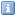#last update
02:30 UTC
SFI: 70= | Ap index:  12  | Kp index:  2  ( n\a @--:--,  n\a @--:--)| X-ray flux:  A7.8
SW: Density= 4.1 p/cm3 | Speed= 409.1 km/s | Temp=38(x1000)K
IMF: Bx=-2.4nT | By=4.7nT | Bz= -1.9 nT | Bt= 5.7 nT | Lat= -20.0 ° | Lon=117.3°
Sunspots: 0 | Area: 0 10-6/Hemi | New regions: 0X-ray flares: C=0 M=0 X=0 | Optical flares: M1=0 M2=0 M3=0 | Sub-flares=0